# Equilibrium Formula for Pricing Commodity Forwards

The forward price of a commodity is calculated much along the lines of that of a financial product except for the fact that by virtue of its physical form and characteristics a commodity incurs different types of storage and holding costs as compared to a financial product and the factors that affect the commodities forward price are much different from those that affect the price of a financial forward.

## The Equations

The equilibrium formula for the calculation of the forward price of a commodity is as follows: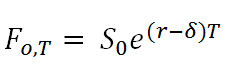Where r is the risk-free interest rate in the market, δ is the lease rate and T is the time between the current date and the future date at which the transaction is supposed to take place.

In financial markets the lease rate is associated with the rate at which returns have been obtained for owning the asset i.e. the dividend yield.

In the commodities market the lease rate arises because the asset can be loaned out in the market as each and every commodity has physical characteristics.

Let’s assume that g is the rate at which the commodity price grows and α is expected rate of return on the commodity price. The lease rate is represented by the following equation: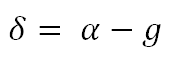There is another way of representing the lease rate. When it is lent out storage costs are saved but the benefits of holding the commodity i.e. the convenience yield is forgone. The borrower of the commodity pays a lease rate that is equal to the convenience yield minus the storage costs.

Let us assume that c is the convenience yield and λ are the storage costs. The lease rate is given by: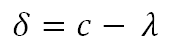This is also called the cost of carrying the commodity.

## The Derivation

If the actual storage cost of the commodity is eλT where λ is the storage cost rate and T is time to maturity then the storage cost can be derived from the spot prices in the market and the prices shown by the warehouses. If the futures price is such that: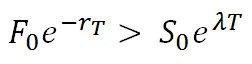The rate in the exponential on the left is the risk-free rate for discounting. A trader might then borrow an amount of money on the right hand side, purchase the commodity and pay for the storage upfront and enter into a short position in the future at time T. Given that all this is satisfied the cashflow is as follows: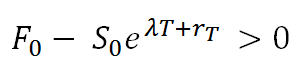There is a possibility of arbitrage in this scheme of things so trade that happens would be one that is of the highest quantity available in the market such that there is a pressure on the movement of the prices such that there is a situation where making risk-free profit does not exist.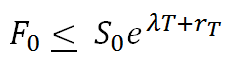If the above inequality is to hold only companies that can sell it and repurchase at a later date because it is not possible to hold negative amounts of commodities and companies do this due to strict industrial requirements. This difference often comes from other industry related requirements and it is modeled via a convenience yield termed as c.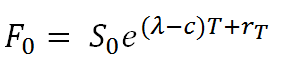# Lease Rate vs. Convenience Yield

The lease rate is the rate that the borrower pays for the commodity he has taken as loan from the lender. The forward price of the commodity is inversely related to the lease rate much like the dividend yield for financial products. The same holds for the relationship between the forward price and the convenience yield as is implied from the formula.

While the cost to the borrower of the commodity is represented by the lease rate the convenience yield is the benefits that the borrower gains during the loan period as a result of possession of the commodity. So the lease rate means something very different than the convenience yield for the commodity.

Get smart about tech at work.

As a non-technical professional, learn how software works with simple explanations of tech concepts. Learn more...

# Data Science for Finance Bundle: 43% OFF

Get our Data Science for Finance Bundle for just $29$51.